×#### Thank you for registering.

One of our academic counsellors will contact you within 1 working day.

Click to Chat

1800-1023-196

+91-120-4616500

CART 0

• 0

MY CART (5)

Use Coupon: CART20 and get 20% off on all online Study Material

ITEM
DETAILS
MRP
DISCOUNT
FINAL PRICE
Total Price: Rs.

There are no items in this cart.
Continue Shopping• Complete JEE Main/Advanced Course and Test Series
• OFFERED PRICE: Rs. 15,900
• View Details

```Chapter 19: Surface Area And Volume of a Right Circular Cylinder Exercise – 19.2

Question: 1

A soft drink is available in two packs-

(i) a tin can with a rectangular base of length 5 cm and width 4 cm, having a height of 15 cm and

(ii) a plastic cylinder with circular base of diameter 7 cm and height 10 cm, Which container has greater capacity and by how much?

Solution:

Given,

The tin can will be cubical in shape.

Length (L) of tin can = 5 cm

Breadth (B) of tin can = 4 cm

Height (H) of tin can = 15 cm

Capacity of the tin can = l × b × h = (5 × 4 x 15) cm3

Radius (R) of the circular end of the plastic cylinder = 7/2 cm = 3.5 cm

Height (H) of plastic cylinder = 10 cm

Capacity of plastic cylinder = πR2H = 22/7 × (3.5)2 × 10 cm3 = 385 cm3

Therefore, the plastic cylinder has greater capacity.

Difference in capacity = (385 - 300) cm3 = 85 cm3

Question: 2

The pillars of a temple are cylindrically shaped. If each pillar has a circular base of radius 20 cm and height 10 m, how much concrete mixture would be required to build 14 such pillars?

Solution:

Given,

The concrete mixture is used to build up the pillars is required for the entire space of the pillar i.e, we need to find the volume of the cylinders.

Radius of the base of a cylinder = 20 cm

Volume of the cylindrical pillar = πR2H

= (22/7 × 202 × 1000) cm3

= 8800000/7 cm3

= 8.8/7 m3 [1m = 100 cm]

Therefore, volume of 14 pillars = 8.8/7 × 14 m3 = 17.6 m3

Question: 3

The inner diameter of a cylindrical wooden pipe is 24 cm and its outer diameter is 28 cm. The length of the pipe is 35 cm. Find the mass of the pipe, if 1 cm3 of wood has a mass of 0.6 gm.

Solution:

Given,

Inner radius (r1) of a cylindrical pipe = 24/2 = 12 cm

Outer radius (r2) of a cylindrical pipe = 24/2 = 14 cm

Height of pipe (h) = length of pipe = 35 cm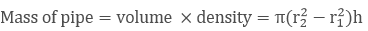= 22/7(142 − 122)35 = 5720 cm3

Mass of 1 cm3 wood = 0.6 gm

Therefore, mass of 5720 cm3 wood = 5720 × 0.6 = 3432 gm = 3.432 kg

Question: 4

If the lateral surface of a cylinder is 94.2 cm2 and its height is 5 cm, find:

(ii) volume of the cylinder [Use pi = 3.141]

Solution:

(i) Given,

Height of the cylinder = 5 cm

Let radius of cylinder be 'r'

Curved surface of the cylinder = 94.2 cm2

2 πrh = 94.2 cm2

r = 3 cm   [π = 31.4, h = 5 cm]

(ii) Volume of the cylinder = πr2h = (3.14 × 32 × 5) cm3 = 141.3 cm3

Question: 5

The capacity of a closed cylindrical vessel of height 1 m is 15.4 liters. How many square meters of the metal sheet would be needed to make it?

Solution:

Given,

Height of the cylindrical vessel = 15.4 litres = 0.0154 m3 [1m3 = 1000 litres]

Let the radius of the circular ends of the cylinders be 'r'

πr2h = 0.0154 m3

r = 0.07 m      [π = 31.4, h = 1m]

Total surface area of a vessel = 2πr(r + h)

= 2(3.14(0.07)(0.07 +1)) m2 = 0.4703 m2

Question: 6

A patient in a hospital is given soup daily in a cylindrical bowl of diameter 7 cm. If the bowl is filled with soup to a height of 4 cm, how much soup the hospital has to prepare daily to serve 250 patients?

Solution:

Given,

Radius (R) of cylindrical bowl = 7/2 cm = 3.5 cm [Diameter = 7 cm]

Height up to which the bowl is filled with soup = 4 cm

Volume of soup in 1 bowl = πr2h

= 22/7 × 3.52 × 4 = 154 cm3

Volume of soup n 250 bowls = (250 × 154) cm3 = 38500 cm3 = 38.5 liters.

The hospital has to prepare 38.5 liters of soup daily in order to serve 250 patients.

Question: 7

A hollow garden roller, 63 cm wide with a girth of 440 cm, is made of 4 cm thick iron. Find the volume of the iron.

Solution:

Given, h = 63 cm

The outer circumference of the roller = 440 cm

Thickness of the roller = 4 cm

Let, R be the external radius

We know that,

22/7 * R = 440

2 * 22/7 * R = 440

R = 70

The thickness is given as 4cm, so the inner radius 'r' is given as

⟹ r = R - 4

⟹ 70 - 4

⟹ 66cm

Here, we know the inner and outer radii

So, the volume is given as

⟹ V = π (R2 − r2)h

= 22/7 * (702 − 662) * 63

= 22/7 * 4 * 136 * 63

= 107712 cm3

Question: 8

A solid cylinder has a total surface area of 231cm2. Its curved surface area is 3 of the total surface area. Find the volume of the cylinder.

Solution:

Given,

Total surface area = 231cm2

Curved surface area = 2/3 * (total surface area)

= 2/3 * 231

= 154

We know that,

2πrh + 2πr2 = 231 ....1

Here 2πrh is the curved surface area, so substitute the value of CSA in eq 1

⟹ 154 + 2πr2 = 231

⟹ 2πr2 = 231- 154

⟹ 2πr2 = 77

⟹ 2 * 22/7 * r2 = 77⟹ r = 7/2

We need to find the value of h

CSA = 154 cm2

⟹ 2πrh = 154

⟹ 2 * 22/7 * 7/2 * h = 154

⟹ h = 154/22

⟹ h = 7

So the volume of the cylinder is,

Volume = πr2h

= 22/7 * 7/2 * 7/2 * 7

= 269.5 cm3

The volume of the cylinder is 269.5 cm3

Question: 9

The cost of painting the total outside surface of a closed cylindrical oil tank at 50 paise per square decimetre is Rs 198. The height of the tank is 6 times the radius of the base of the tank. Find the volume corrected to 2 decimal places.

Solution:

Let the radius of the tank be r dm

Then, height = 6r dm

Cost of painting for 50 paisa per dm2 = Rs 198

⟹ 2πr(r + h) = 198

⟹ 2 × 22/7 × r(r + 6r) × 1/2 = 198

⟹ r = 3 dm

Therefore, h = (6 × 3) dm = 18 dm

Volume of the tank = πr2h = 22/7 × 9 × 18 = 509.14 dm3

Question: 10

The radii of two cylinders are in the ratio 2:3 and their heights are in the ratio 5:3. Calculate the ratio of their volumes and the ratio of their curved surfaces.

Solution:

Let the radius of the cylinders be 2× and 3× and the height of the cylinders be 5y and 3y.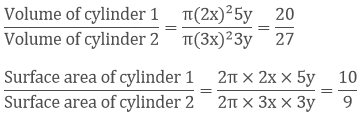Question: 11

The ratio between the curved surface area and the total surface area of a right circular cylinder is 1: 2. Find the volume of the cylinder, if its total surface area is 616 cm2.

Solution:

Let, r be the radius of cylinder

h be the radius of cylinder

Total surface area (T.S.A) = 616 cm2⟹ CSA = 1/2 * TSA

⟹ CSA = 1/2 * 616

⟹ CSA = 308 cm2

Now,

TSA = 2πrh + 2πr2

⟹ 616 = CSA + 2πr2

⟹ 616 = 308 + 2πr2

⟹ 2πr2 = 616 – 308

⟹ 2πr2 = 308

⟹ πr2 = 308/2

⟹ r2 = 308/2π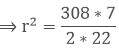⟹ r = 7 cm

Since, CSA = 308 cm2

⟹ 2πrh = 308

⟹ 2 * 22/7 * 7 * h = 308

⟹ h = 7cm

Volume of cylinder = πr2 * h

= 22/7 * 7 * 7 * 7

= 22 * 49 = 1078 cm2

Question: 12

The curved surface area of a cylinder is 1320 cm2 and its base had diameter 21 cm. Find the height and volume of the cylinder.

Solution:

Let, r be the radius of the cylinder

h be the height of the cylinder

⟹ 2r = 21cm

⟹ r = 21/2

= 10.5 cm

Given, Curved surface area (CSA) = 1320 cm2

⟹ 2πrh = 1320

⟹ 2 * 22/7 * 10.5 * h = 1320

⟹ h = 1320/66

⟹ h = 20 cm

Volume of cylinder = πr2 * h

= 22/7 * 10.5 * 10.5 * 20

= 22 * 1.5 * 10.5 * 20

= 6930 cm2

Question: 13

The ratio between the radius of the base and the height of a cylinder is 2:3. Find the total surface area of the cylinder, if its volume is 1617 cm2.

Solution:

Let, r be the radius of the cylinder

h be the height of the cylinder

r/h = 2/3

r = 2/3 * h  ....1

Volume of cylinder = πr2 * h

1617 = 22/7 *(2/3 ∗ h)2 * h

1617 = 22/7 * (2/3 ∗ h)3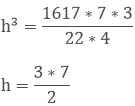h = 10.5 cm

from, eq 1

r = 2/3 * 10.5

= 7 cm

Total surface area of cylinder = 2πr (h + r)

= 2 * 22/7 * 7(10.5 + 7)

= 44 * 17.5 = 770 cm3

Question: 14

A rectangular sheet of paper, 44 cm * 20 cm, is rolled along its length of form cylinder. Find the volume of the cylinder so formed.

Solution:

Given, the dimensions of the sheet are 44 cm * 20 cm

Here, length = 44 cm

Height = 20 cm

2πr = 44

r = 44/2π

r = 44/2π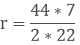r = 7 cm

Volume of cylinder = r2 * h

= 22/7 * 7 * 7 * 20

= 154 * 20 = 3080 cm3

Question: 15

The curved surface area of cylindrical pillar is 264 m2 and its volume is 924 m3. Find the diameter and the height of the pillar.

Solution:

Let, r be the radius of the cylindrical pillar

h be the height of the cylindrical pillar

CSA = 264 m2

2πrh = 264m2  ....1

⟹ Volume of the cylinder = 924 m2

Π * r2 * h = 924

πrh(r) = 924

πrh = 924/r

Substitute πrh in eq 1

2 * 924/r = 264

r = 1848/264

r = 7 m

Substitute r value in eq 1

2 * 22/7 * 7 * h = 264

h = 264/44

h = 6 m

so, the diameter = 2r = 2(7) = 14 m and height = 6 m

Question: 16

Two circular cylinders of equal volumes have their heights in the ratio 1:2. Find the ratio of two radii.

Solution:

Let, r1, r2 be the radii of the cylinder

h1, h2 be the height of the cylinder

v1, v2 be the volume of the cylinder

h1/h2 = 1/2 and v1 = v2

⟹ v1/v2 = (r1/r2)2 * (h1/h2)

Since, v1 = v2

⟹ v1/v1 = (r1/r2)2 * (1/2)

⟹ 1 = (r1/r2)2 * (1/2)

⟹ (r1/r2)2 = (2/1)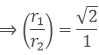Hence, the ratio of the radii are √2:1

Question: 17

The height of a right circular cylinder is 10.5 m. Three times the sum of the areas of its two circular faces is twice the area of the curved surface. Find the volume of the cylinder.

Solution:

Let, r be the radius of the right circular cylinder

h be the height of the right circular cylinder

h = 10.5 cm

⟹ 3(2πr2) = 2(2πrh)

⟹ 3r = 2h

⟹ r = 2/3 ∗ h

⟹ r = 2/3 ∗ 10.5

⟹ r = 7cm

Volume of the cylinder = r2 * h

= 22/7 * 7 * 7 * 10.5

= 154 * 10.5

= 1617 cm3

Question: 18

How many cubic meters of earth must be dug out to sink a well 21m deep and 6 m diameter? Find the cost of plastering the inner surface as well at Rs.9.50 per m2.

Solution:

h be the height

here, h = 21m

2r = 6

⟹ r = 6/2

= 3 m

Volume of the cylinder = r2 * h

= 22/7 * 3 * 3 * 21

= 66 * 9

= 594 cm3

Cost of plastering = 9.5 per m3

Cost of plastering inner surface = Rs.(594 * 9.50) = Rs. 5643

Question: 19

The trunk of a tree is cylindrical and its circumference is 176 cm. If the length of the tree is 3 m. Find the volume of the timber that can be obtained from the trunk.

Solution:

We know that, circumference = 2πr

⟹ 176 = 2πr

⟹ r = 176/2π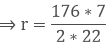⟹ r = 28 cm

Here, height (h) = 3 m = 300 cm

Volume of timber = r2 * h

= 22/7 * 28 * 28 * 300

= 44 * 8400 = 739200 cm3 (or) 0.7392 m3

Question: 20

A well with 14 m diameter is dug 8 m deep. The earth taken out of it has been evenly spread all around it to a width of 21 m to form an embankment. Find the height of the embankment.

Solution:

Let, r be the radius of well

h be the height of well

here, h = 8 m

2r = 14

⟹ r = 14/2

= 7m

Volume of well = r2 * h

= 22/7 * 7 * 7 * 8

= 22 * 56

= 1232 m3

Let, re be the radius of embankment

he be the height of embankment

Volume of well = Volume of embankment

1232 m3 = π ∗ re ∗ he

1232 = 22/7 ∗ (282 − 72) ∗ he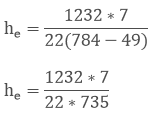he = 0.533 m

Question: 21

The difference between inside and outside surfaces of a cylindrical tube is 14 cm long is 88 sq.cm. If the volume of the tube is 176 cubic cm, Find the inner and outer radii of the tube.

Solution:

Let, R be the outer radius

Here, h = 14 cm

2πRh - 2πrh = 88

⟹ 2πh(R – r) = 88

⟹ 2 * 22/7 * 14(R – r) = 88

⟹ (R – r) = 1cm .....1

Volume of tube = π * R2 * h – π * r2 * h

176 = πh(R2 - r2)

176 = 22/7 *14(R2 - r2)

⟹ (R2 - r2) = 4

⟹ (R + r)(R – r) = 4

Here, (R - r) = 1

⟹ (R + r) (1) = 4

⟹ (R + r) = 4 cm

⟹ R = 4 – r  ....2

Here, R – r = 1

⟹ R = 1 + r

Substitute R value in eq 2

⟹ 1 + r = 4 – r

⟹ 2r = 3

⟹ r = 3/2

= 1.5 cm

Substitute ‘r’ value in eq 1

⟹ R – 1.5 = 1

⟹ R = 1 + 1.5

⟹ R = 2.5 cm

Hence, the value of inner radii is 1.5 cm and radius of outer radii is 2.5 cm

Question: 22

Water flows out through a circular pipe whose internal diameter is 2 cm, at the rate of 6 meters per second into a cylindrical tank. The water is collected in a cylindrical vessel radius of whose base is 60 cm. Find the rise in the level of water in 30 minutes?

Solution:

Given data is as follows:

Internal diameter of the pipe = 2 cm

Water flow rate through the pipe = 6 m/sec

Radius of the tank = 60 cm

Time = 30 minutes

The volume of water that flows for 1 sec through the pipe at the rate of 6 m/sec is nothing but the volume of the cylinder with n = 6

Also, given is the diameter which is 2 cm. Therefore,

R = 1 cm

Since the speed with which water flows through the pipe is in meters/second, let us convert the radius of the pipe from centimeters to meters. Therefore,

r = 1/100 m

Volume of water that flows for 1 sec = 22/7 × 1/100 × 1/100 × 6

Now, we have to find the volume of water that flows for 30 minutes.

Since, speed of water is in metres/second, let us convert 30 minutes into seconds. It will be 30 × 60

Volume of water that flows for 30 minutes = 22/7 × 1/100 × 1/100 × 6 × 30 × 60

Now, considering the tank, we have been given the radius of tank in centimeters. Let us first convert it into metres. Let radius of tank be ‘R’.

R = 60 cm

R = 60/100 m

Volume of water collected in the tank after 30 minutes = Volume of water that flows through the pipe for 30 minutes

22/7 × 60/100 × 60/100 × h = 22/7 × 1/100 × 1/100 × 6 × 30 × 60

h = 3 m

Therefore, the height of the tank is 3 metres.

Question: 23

A cylindrical container with diameter of base 56 cm contains sufficient water to submerge a rectangular solid of iron with dimensions 32 cm × 22 cm × 14 cm. Find the rise in the level of the water when the solid is completely submerged.

Solution:

Given data is as follows:

Diameter of cylinder = 56 cm

Dimensions of rectangular block = 32 cm × 22 cm × 14 cm

We have to find the raise in the level of water in the cylinder.

First let us find the raise in the level of water in the cylinder. Diameter is given as 56 cm. Therefore,

r = 28 cm

We know that the raise in the volume of water displaced in the cylinder will be equal to the volume of the rectangular block.

Let the raise in the level of water be h. Then we have,

Volume of cylinder of height h and radius 28 cm = Volume of the rectangular block

22/7 × 28 × 28 × h = 32 × 22 × 14

h = 4 cm

Therefore, the raise in the level of water when the rectangular block is immersed in the cylinder is 4 cm.

Question: 24

A cylindrical tube, open at both ends, is made of metal. The internal diameter of the tube is 10.4 cm and its length is 25 cm. The thickness of the metal is 8 mm everywhere. Calculate the volume of the metal.

Solution:

Given data is as follows:

Internal diameter = 10.4 cm

Thickness of the metal = 8 mm

Length of the pipe = 25 cm

We have to find the volume of the metal used in the pipe.

We know that,

Volume of the hollow pipe = π(R2 − r2)h

Given is the internal diameter which is equal to 10.4 cm .Therefore,

r = 10.4/2

r = 5.2 cm

Also, thickness is given as 8 mm. Let us convert it to centimeters.

Thickness = 0.8 cm

Now that we know the internal radius and the thickness of the pipe, we can easily find external radius 'R'.

R = 5.2 + 0.8

R = 6 cm

Therefore, Volume of metal in the pipe = 22/7 × (62 − 5.22) × 25

= 704 cm3

Therefore, the volume of metal present in the hollow pipe is 704 cm3.

Question: 25

From a tap of inner radius 0.75 cm, water flows at the rate of 7 m per second. Find the volume in litres of water delivered by the pipe in one hour.

Solution:

Given data is as follows:

r = 0.75 cm

Water flow rate = 7 m/sec

Time = 1 hour

We have to find the volume of water that flows through the pipe for 1 hour.

Let us first convert water flow from m/sec to cm/sec, since radius of the pipe is in centimeters

We have,

Water flow rate = 7 m/sec

= 700 cm/sec

Volume of water delivered by the pipe is equal to the volume of a cylinder with h = 7 m and r = 0.75 cm. Therefore,

Volume of water delivered in 1 second = 22/7 × 0.75 × 0.75 × 700

We have to find the volume of water delivered in 1hour which is nothing but 3600 seconds.

Therefore, we have

Volume of water delivered in 3600 seconds = 22/7 × 0.75 × 0.75 × 700 × 3600 = 4455000 cm3.

We know that 1000 cm3 = 1 litre

Therefore,

Volume of water delivered in 1 hour = 4455 liters

Therefore, Volume of water delivered by the pipe in 1 hour is equal to 4455 liters.

Question: 26

A cylindrical water tank of diameter 1.4 m and height 2.1 m is being fed by a pipe of diameter 3.5 cm through which water flows at the rate of 2 metre per second. In how much time the tank will be filled?

Solution:

Given data is as follows:

Diameter of the tank = 1.4 m

Height of the tank = 2.1 m

Diameter of the pipe = 3.5 cm

Water flow rate = 2 m/sec

We have to find the time required to fill the tank using the pipe.

The diameter of the tank is given which is 1.4 m. Let us find the radius.

r = 1.4/2 = 0.7 m

Volume of the tank = πr2h

= 22/7 × 0.7 × 0.7 × 2.1

Given is the diameter of the pipe which is 3.5 cm. Therefore, radius is 3.5/2 cm. Let us convert it into metres. It then becomes, 3.5/200 m.

Volume of water that flows through the pipe in 1 second = 22/7 × 3.5/200 × 3.5/200 × 2

Let the time taken to fill the tank be x seconds. Then we have,

Volume of water that flows through the pipe in x seconds = 22/7 × 3.5/200 × 3.5/200 × 2 × x

We know that volume of the water that flows through the pipe in x seconds will be equal to the volume of the tank. Therefore, we have

Volume of water that flows through the pipe in x seconds = Volume of the tank

22/7 × 3.5/200 × 3.5/200 × 2 × x = 22/7 × 0.7 × 0.7 × 2.1

x = 1680 seconds

x = 1680/60 minutes

x = 28 minutes

Hence, it takes 28 minutes to fill the tank using the given pipe.

Question: 27

A rectangular sheet of paper 30 cm × 18 cm can be transformed into the curved surface of a right circular cylinder in two ways i.e. either by rolling the paper along its length or by rolling it along its breadth. Find the ratio of the volumes of the two cylinders thus formed.

Solution:

Given data is as follows:

Dimensions of the rectangular sheet of paper = 30 cm × 18 cm

We have to find the ratio of the volumes of the cylinders formed by rolling the sheet along its length and along its breadth.

Let V1 be the volume of the cylinder which is formed by rolling the sheet along its length.

When the sheet is rolled along its length, the length of the sheet forms the perimeter of the cylinder. Therefore, we have,

2πr1 = 30

r1 = 15/π

The width of the sheet will be equal to the height of the cylinder. Therefore,

h1 = 18 cm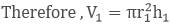= π × 15/π × 15/π × 18

V1 = 225/π × 18 cm3

Let V2 be the volume of the cylinder formed by rolling the sheet along its width.

When the sheet is rolled along its width, the width of the sheet forms the perimeter of the base of the cylinder. Therefore, we have,

2πr2 = 18

r2 = 9/π

The length of the sheet will be equal to the height of the cylinder. Therefore,

h2 = 30 cm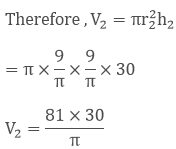Now that we have the volumes of two cylinders, we have,Therefore, the ratio of the volumes of the two cylinders is 5: 3.

Question: 28

How many litres of water flow out of a pipe having an area of cross-section of 5 cm2 in one minute, if the speed of water in the pipe is 30 cm/sec ?

Solution:

Given data is as follows:

Area of cross section of the pipe = 5 cm2

Speed of water = 30 cm/sec

We have to find the volume of water that flows through the pipe in 1 minute.

Volume of water that flows through the pipe in one second = πr2h

Here, πr2 is nothing but the cross section of the pipe and h is 30 cm.

Therefore, we have,

Volume of water that flows through the pipe in one second = 5 × 30 = 150 cm3

Volume of water that flows through the pipe in one minute = 150 × 60 = 9000 cm3

We know that 1000 cm3 = 1 litre. Therefore,

Volume of water that flows through the pipe in one minute = 9 litres

Hence, the volume of water that flows through the given pipe in 1 minute is 9 litres.

Question: 29

The sum of the radius of the base and height of a solid cylinder is 37 m. If the total surface area of the solid cylinder is 1628 cm2. Find the volume of the cylinder.

Solution:

Given data is as follows:

h + r = 37 cm

Total surface area of the cylinder = 1628 cm2

That is,

2πrh + 2πr2 = 1628

2πr(h + 2r) = 1628

But it is already given in the problem that,

h + r = 37 cm

Therefore, 2πr × 37 = 1628

2 × 22/7 × r × 37 = 1628

r = 7 cm

Since, h + r = 37 cm

We have, h + 7 = 37 cm

H = 30 cm

Now that we know both height and radius of the cylinder, we can easily find the volume.

Volume = πr2h

Volume = 22/7 × 7 × 7 × 30

Volume = 4620 cm3

Hence, the volume of the given cylinder is 4620 cm3.

Question: 30

Find the cost of sinking a tube well 280 m deep, having diameter 3 m at the rate of Rs 3.60 per cubic metre. Find also the cost of cementing its inner curved surface at Rs 2.50 per square metre.

Solution:

Given data is as follows:

Height of the tube well = 280 m

Diameter = 3 m

Rate of sinking of the tube well = Rs. 3.60/m3

Rate of cementing = Rs. 2.50/m2

Given is the diameter of the tub well which is 3 metres. Therefore,

r = 3/2 m

Volume of the tube well = πr2h

= 22/7 × 3/2 × 3/2 × 280

= 1980 m2

Cost of sinking the tube well = Volume of the tube well × Rate of sinking the tube well = 1980 × 3.60

= Rs. 7128

Curved surface area = 2πrh

= 2 × 22/7 × 3/2 × 280

= 2640 m2

Cost of cementing = Curved Surface area × Rate of cementing

= 2640 × 2.50

= Rs. 6600

Therefore, the total cost of sinking the tube well is Rs.7128 and the total cost of cementing its inner surface is Rs. 6600.

Question: 31

Find the length of 13.2 kg of copper wire of diameter 4 mm, when 1 cubic cm of copper weighs 8.4 gm.

Solution:

Given data is as follows:

Weight of copper wire = 13.2 kg

Diameter = 4 mm

Density = 8.4 gm /cm3

We have to find the length of the copper wire.

Given is the diameter of the wire which is 4 mm. Therefore,

r = 2 mm

Let us convert r from millimeter to centimeter, since density is in terms of gm/cm3. Therefore,

r = 210 cm

Also, weight of the copper wire is given in kilograms. Let us convert into grams since density is in terms of gm/cm3. Therefore, we have,

Weight of copper wire = 13.2 × 1000 gm

= 13200 gm

We know that

Volume × Density = Weight

Therefore, πr2h × 8.4 = 13.2

22/7 × 2/10 × 2/10 × h × 8 .4

h = 12500 cm

h = 125 m

Hence, the length of the copper wire is 125 metres.

Question: 32

A well with 10 m inside diameter is dug 8.4 m deep. Earth taken out of it is spread all around it to a width of 7.5 m to form an embankment. Find the height of the embankment.

Solution:

Given data is as follows:

Inner diameter of the well = 10 m

Height = 8.4 m

Width of embankment = 7.5 m

We have to find the height of the embankment.

Given is the diameter of the well which is 10 m. Therefore,

r = 5 m

The outer radius of the embankment,

R = Inner radius of the well + width of the embankment

= 5 + 7.5

= 12.5 m

Let H be the height of the embankment.

The volume of earth dug out is equal to the volume of the embankment. Therefore,

Volume of embankment = Volume of earth dug out

π(R2 − r2)H = πr2h

22/7 × (12.52 − 52)H = 22/7 × 5 × 5 × 8.4

H = 1.6 m

Thus, height of the embankment is 1.6 m.
```### Course Features

• 728 Video Lectures
• Revision Notes
• Previous Year Papers
• Mind Map
• Study Planner
• NCERT Solutions
• Discussion Forum
• Test paper with Video Solution